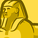# New to Qlik Sense

If you’re new to Qlik Sense, start with this Discussion Board and get up-to-speed quickly.

Announcements
cancel
Showing results for
Did you mean:Contributor III

## Set analysis with 3 conditions

Hi there,

I want to calculate the number of customers with an event name = 'P', the sum of total events >=2, and within the last 12 months.

I am using the following expression, but it does not return any value.

COUNT({<
[Customer ID] = {"=sum({< [Event Type]={'P'},Date={">=\$(=date(addmonths(Max(Date),-12)))<=\$(=date(Max(Date)))"}>}
[Total Events])>=2"}
>}
distinct [Customer ID])

Labels (2)

• ### Set Analysis

9 RepliesMVP

use month and try

COUNT({<
[Customer ID] = {"=sum({< [Event Type]={'P'},Month={">=AddMonths(Max(Month),-12) <=Max(Month)"}>}
[Total Events])>=2"}
>}
distinct [Customer ID])Specialist

First try to create flag in the script for sum(total events) >=2 and then call the flag in the set analysisContributor III
Author

My script like this, If I create a flag as you suggested. I need to use group by and group by date, then that would not work.

"Event Type",
"Customer ID",
"Date",
"Total Events"
FROM [lib://AttachedFiles/94cc618a-ac95-49f8-a660-3e2633e6e067.xlsx]
(ooxml, embedded labels, table is Sheet1);Contributor III
Author

besides I also need to use the total events column for other calculation.SpecialistContributor III
Author

Hi @manoranjan_d , could you please describe in more detail? I am very new to Qlik sense, Thank youSpecialist

do you want to calculate the [Customer ID] who is having sum   [Total Events])>=2? if so

then you link your fact table key -customer id with dimension table.

example:

if(sum(total event >=2,'Y','N')

resident Table

group by customer IDContributor III
Author

Yes but it would not work, I need to not only calculate that but also take into consideration the last 12 months.

Adding that script only gives me the customer who >=2 but in my expression, I also need to add a date filter.

test:

if(sum("Total Events" >=2),'Y','N') AS Flag,
"Customer ID",

Resident Events
group by "Customer ID",

and then linked with my fact table :

COUNT({<
Flag={'Y'}
>} distinct[Customer ID])

it does not return correct resultSpecialist